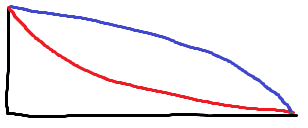# Time falling on slopes of same length

• feynman1
In summary, the conversation discusses using variational calculus and a graphical solution to solve a problem. The time passing a path interval is determined by the length parameter and tangential speed of the path, and conservation of energy is used to relate the tangential speed to the vertical coordinate. The conversation also mentions investigating an integral to find the time for different paths, and concludes with the moderator closing the thread due to the lack of effort from the original poster.

#### feynman1

No Effort shown on schoolwork-type problem
Homework Statement
A ball falls from the top of 2 slopes of the same length as shown. No friction and no assumption of the convexity of either curve (they might be convex and concave at various locations, but the blue is always above the red). Which schematic solution is the best in showing that it falls from the red curve faster?
Relevant Equations
mechanical energy conservedWhat are your thoughts? We cannot help until you have showed some effort yourself.

jbriggs444 said:
What are your thoughts? We cannot help until you have showed some effort yourself.
One can use variational calculus, but I'm looking for a graphical solution.

feynman1 said:
One can use variational calculus, but I'm looking for a graphical solution.
You'd have to figure out what to graph, which may be easier once you have solved it algebraically.

Time passing the path interval [l,l+dl] is
$$dt=\frac{dl}{v(l)}$$
where l is length parameter of the path and v(l) is tangential speed as function of l.
By conservation of energy v(l) = v(y(l)) where y is vertical coordinate of the path and y=0 at start top l=0.

anuttarasammyak said:
Time passing the path interval [l,l+dl] is
$$dt=\frac{dl}{v(l)}$$
where l is length parameter of the path and v(l) is tangential speed as function of l.
By conservation of energy v(l) = v(y(l)) where y is vertical coordinate of the path and y=0 at start top l=0.
But it is not necessarily the case that ##v_{lower}(l)>v_{higher}(l)## for all l.

•nasu and anuttarasammyak
We shall investigate integral
$$T=C \int_0^L \frac{dl}{\sqrt{-y(l)}}$$
where y(0)=0, y(L)=-H.

For path 1 and path 2, in the case ##y_2(l) > y_1(l)## for all l, obviously ##T_2 > T_1##.

Last edited:
Thread closed for Moderation (no effort shown by OP).

OP has received a standard infraction for showing no work on a schoolwork-type question, and since this infraction adds to a number of previous infractions for the OP, this has resulted in a permanent ban. Thread will remain closed.